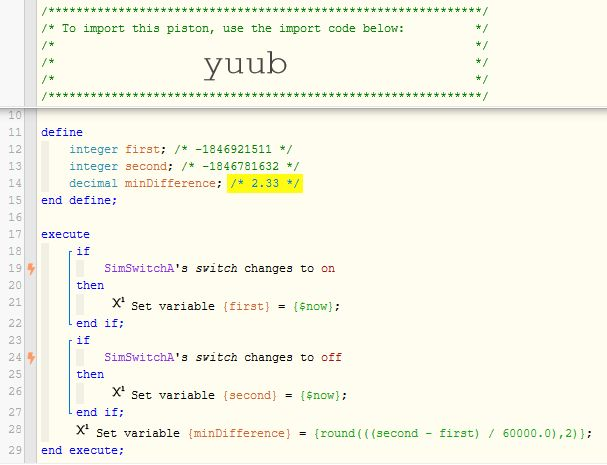# Calculating time between events

#1

THIS POST PERTAINS TO BOTTOM STATEMENT

I figured this wouldn’t work but wanted to at least try. How can I take two times and find the difference between them? Basically want to set a variable to the amount of time between now and a prior event. Then use that variable so that if the amount of time has been less than 120 minutes do one thing but if it’s been over 120 minutes do something else. As I’ve got it now the variable keeps getting set to 0.

#2

Easiest way is to set the start time to \$now. Then use one of the time functions and you can determine if it’s been at least that amount of time or less

if \$now is after addminutes(start_variable,120). There is addsecounds, minutes, hours, days, etc.
The above command would verify it’s been at least 120 minutes.

Look here

#3

I usually dump `\$now` into integer `variables` on both events, and then do the math based on that.
(divide by 1,000 for seconds, or divide by 60,000 for minutes, or divide by ‭3,600,000‬ for hours)

Here is a quick test piston I made to show it in action:The highlighted `variable` is how many minutes passed between the two events.
(On line 28, you can change the “2” to a “0” if you want the minutes rounded to a whole number)

#4

So I went a slightly different route with the expression but it works as far as calculating time. Now my problem is the next step. My goal is to basically say if the amount of time (variable TimeOpen) is over 120 minutes then do X but if it’s less then do Y but no matter what I do it always comes up true or says it’s been longer than TimeOpen.

#5

If you convert to a number first (specifically minutes), it is a piece of cake. (as seen highlighted below)

On line 30, if you want to avoid typos, you can copy/paste this into your `Expression` box:
`round(((second - first) / 60000.0),2)`

#6

Thanks. Just to be sure this comes out in minutes right?

#7

Yes. Dividing by 60,000 is converting milliseconds to minutes.

If the third `variable` is a decimal, (like my example), then it will show two decimals.

#8

\$time is time only whereas \$CurrenteventDate is a date and time format.

Perhaps you should use \$now instead of \$time. I would guess mixing the two might give unexpected results?

#9

I agree. `\$now` gave better results in my testing.

#10

Reason for using \$time was because I’m also going to be announcing the time and \$time just gives me time but \$now gave me date and time.

#11

Okay, but I still think you need to do your calculation using two like formats.

#12

Here’s my final piston. Haven’t fully tested yet. Thanks for all the help so far. Always appreciated.

#14

Any clue why I’m getting a negative number or the E at the end for TimeOpen variable?

#15

Upon further review I see the StatusTimeInt variable isn’t even being set for some reason.

#16

#17

Duh I’m so embarrassed thanks again.

#18

Don’t sweat it… We’ve all done this at one time or another…#19

Been trying to figure something similar out recently, great info all!